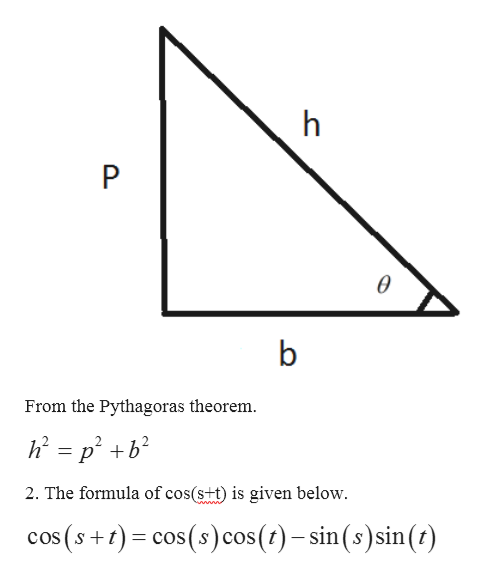# Find the exact value of the expression using the provided information.Find cos(s + t) given that cos s =1/3, with s in quadrant I, and sin t=-1/2  with t in quadrant IV.

Question
3 views

Find the exact value of the expression using the provided information.

Find cos(s + t) given that cos s =1/3, with s in quadrant I, and sin t=-1/2  with t in quadrant IV.

check_circle

Step 1

To determine the value of cos (s + t).

Step 2

Given information:

The trigonometric functions are given below.

Step 3

Used concept:

1. 1. Pythagoras theorem:

Let hypotenuse is h, perpendicular...help_outlineImage Transcriptioncloseh P b From the Pythagoras theorem 2. The formula of cos(s+t) is given below. cos(st) cos(s) cos (t)-sin(s)sin(t) fullscreen

### Want to see the full answer?

See Solution

#### Want to see this answer and more?

Solutions are written by subject experts who are available 24/7. Questions are typically answered within 1 hour.*

See Solution
*Response times may vary by subject and question.
Tagged in

### Trigonometric Ratios